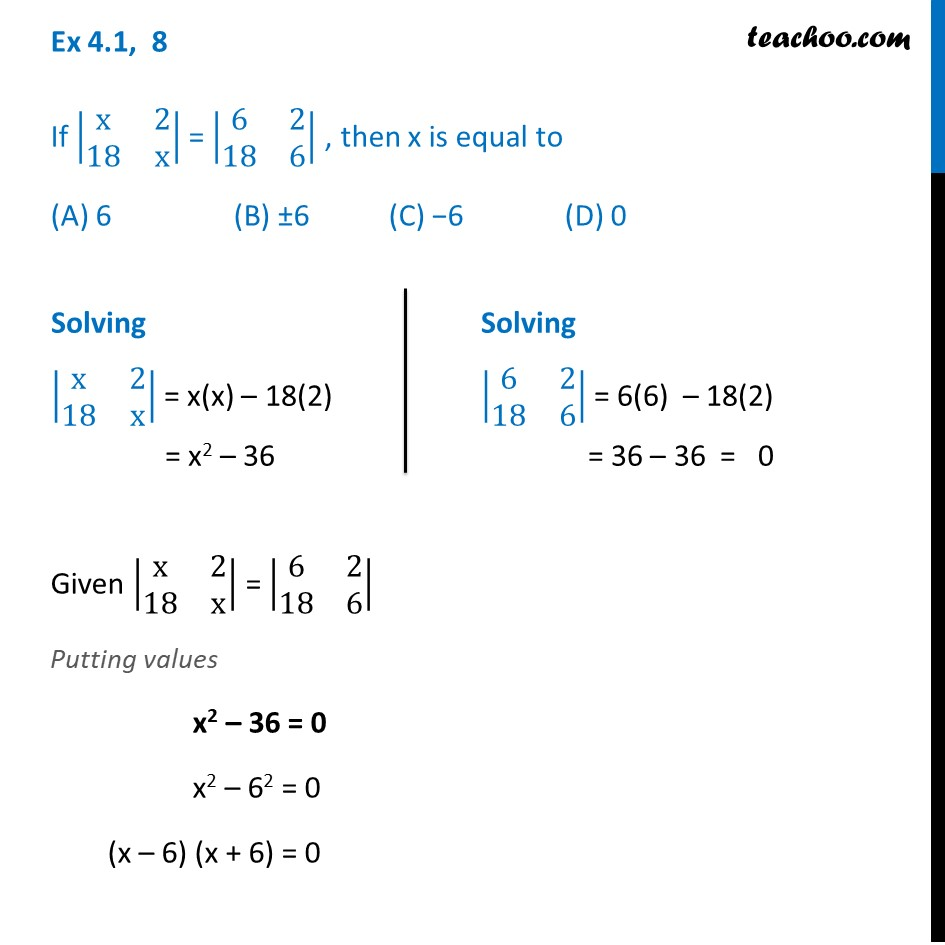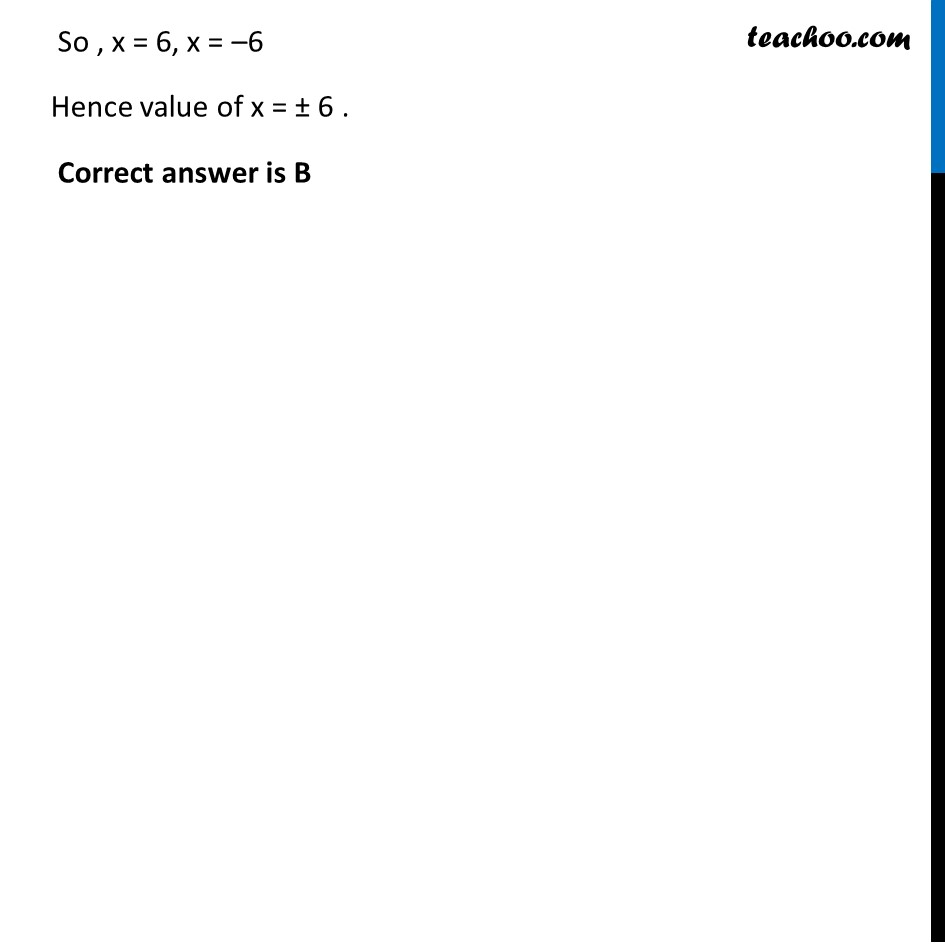Ex 4.1

Chapter 4 Class 12 Determinants
Serial order wiseLearn in your speed, with individual attention - Teachoo Maths 1-on-1 Class

### Transcript

Ex 4.1, 8 If |■8(x&2@18&x)| = |■8(6&2@18&6)| , then x is equal to (A) 6 (B) ±6 (C) −6 (D) 0 Given |■8(x&2@18&x)| = |■8(6&2@18&6)| Putting values x2 – 36 = 0 x2 – 62 = 0 (x – 6) (x + 6) = 0 Solving |■8(x&2@18&x)| = x(x) – 18(2) = x2 – 36 Solving |■8(6&2@18&6)| = 6(6) – 18(2) = 36 – 36 = 0 So , x = 6, x = –6 Hence value of x = ± 6 . Correct answer is B